### IMO Shortlist 2001 problem C1

Kvaliteta:
Avg: 0,0
Težina:
Avg: 5,5
Let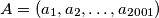$A = (a_1, a_2, \ldots, a_{2001})$ be a sequence of positive integers. Let$m$ be the number of 3-element subsequences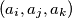$(a_i,a_j,a_k)$ with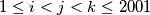$1 \leq i < j < k \leq 2001$, such that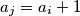$a_j = a_i + 1$ and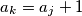$a_k = a_j + 1$. Considering all such sequences$A$, find the greatest value of$m$.
Izvor: Međunarodna matematička olimpijada, shortlist 2001